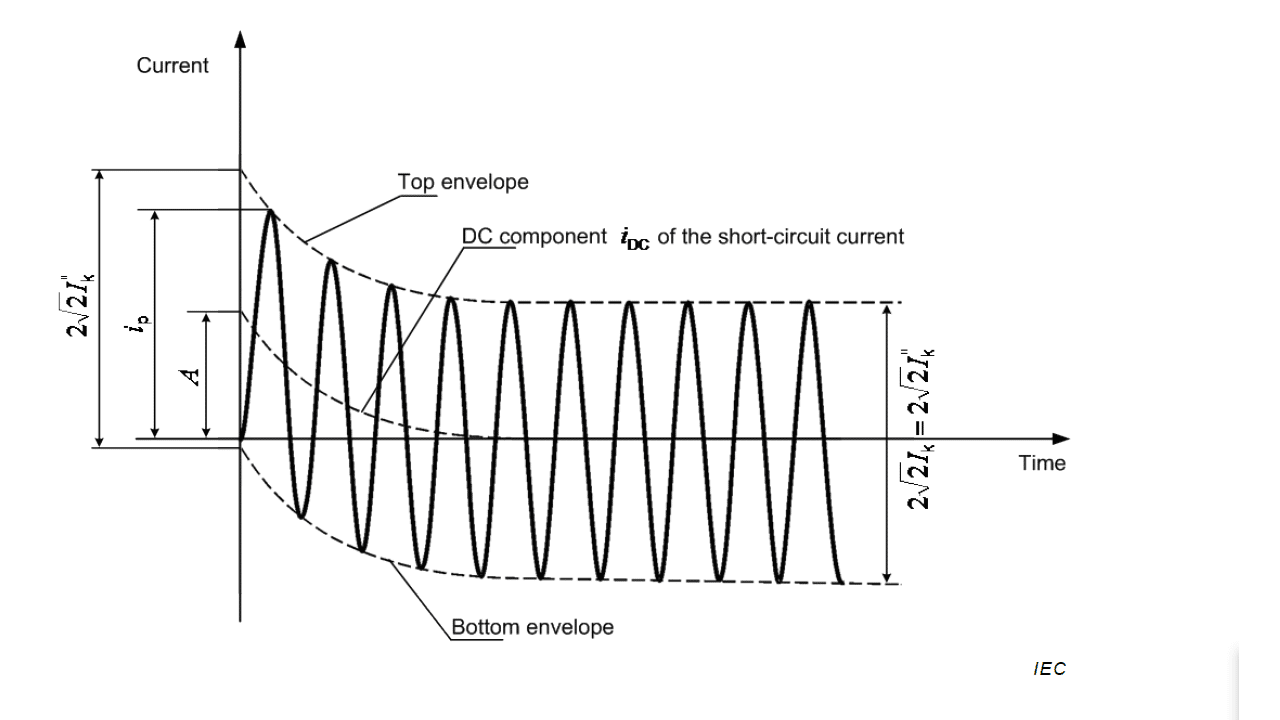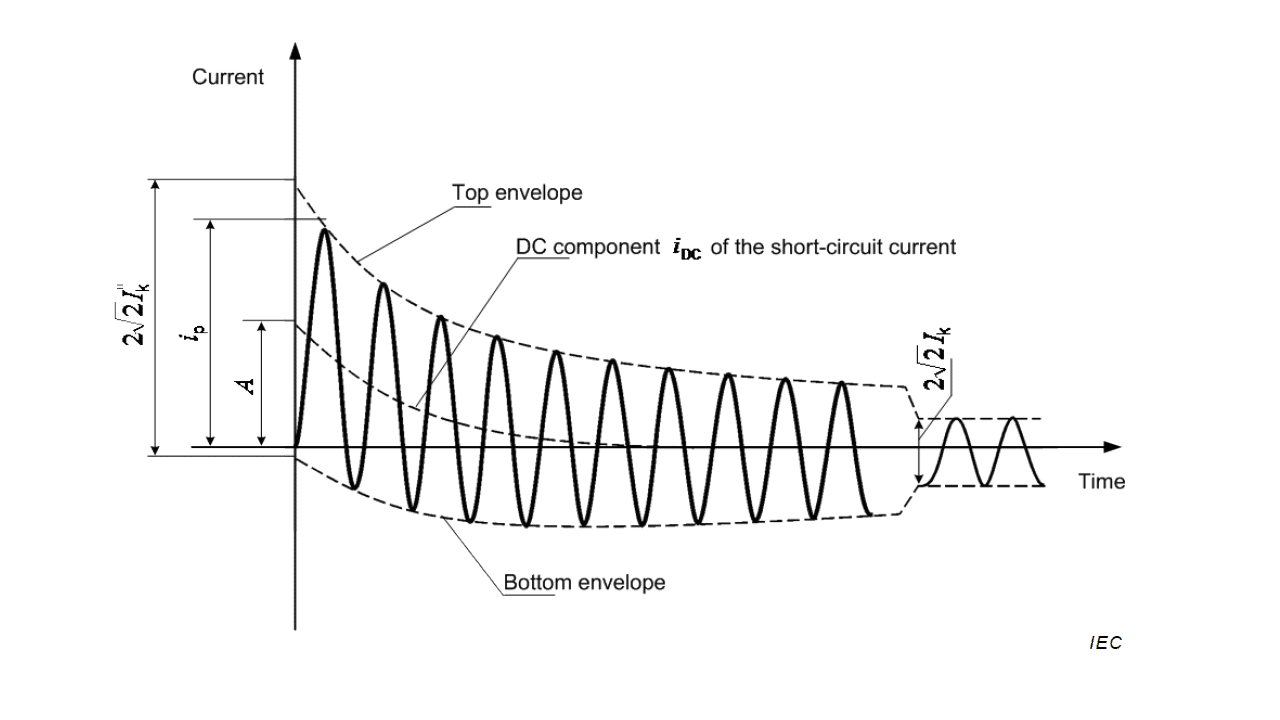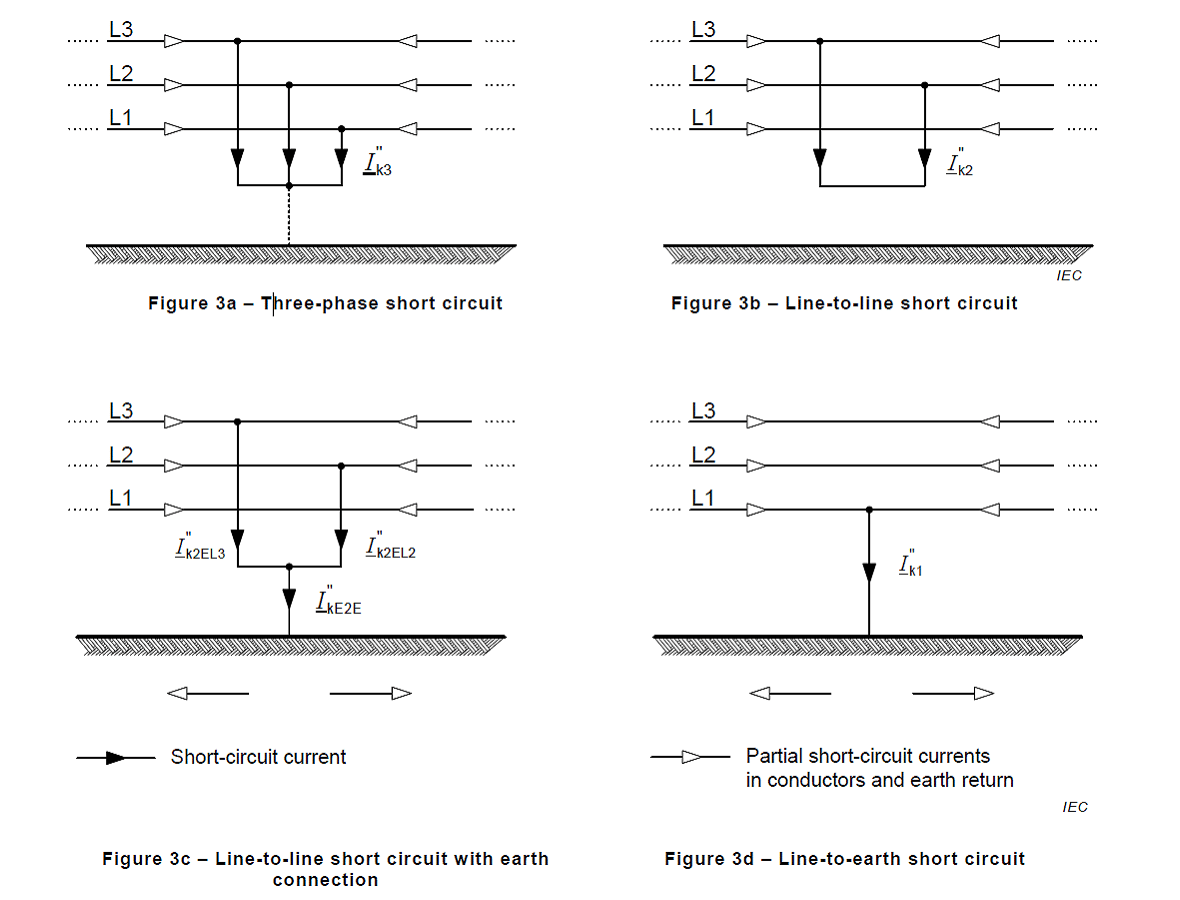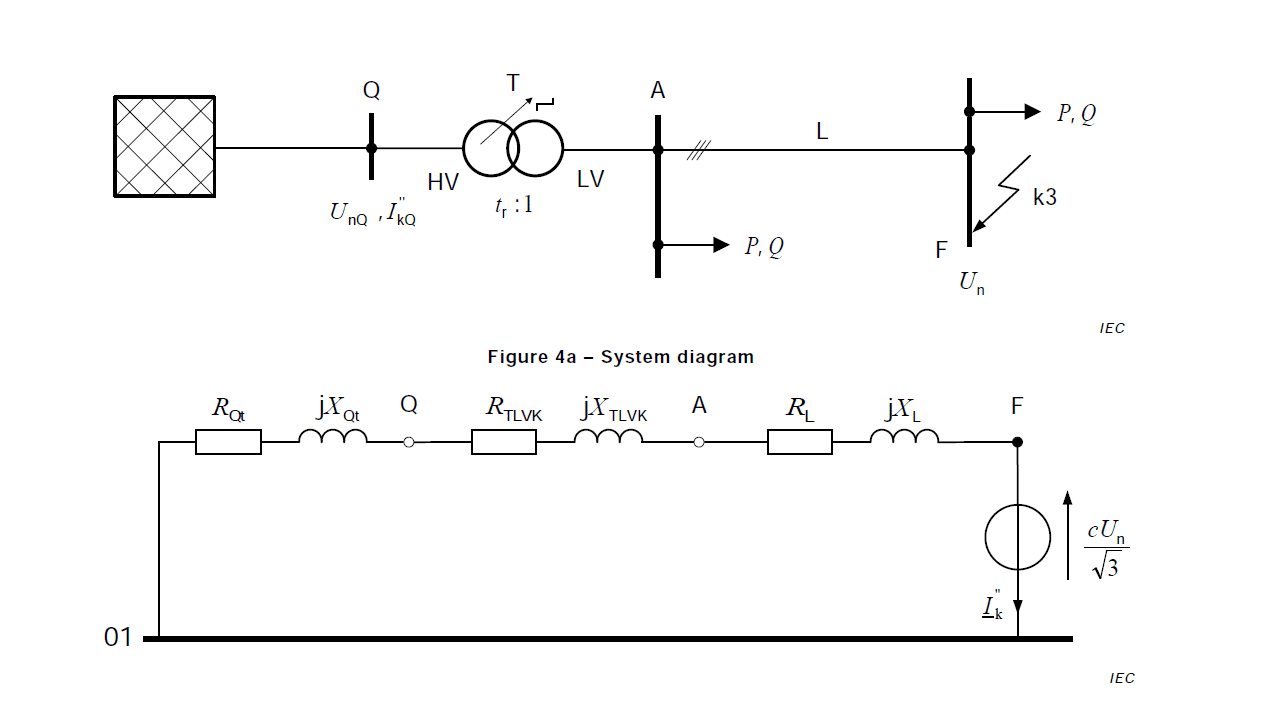# Methods for Short-Circuit Current Calculation

Methods for calculating short-circuit currents are outlined in IEC 60909 standards and technical reports. The IEC 60909 complex is used to calculate short-circuit currents in low-voltage and high-voltage AC power systems with a frequency of 50 or 60 Hz.

### General

A complete calculation of short-circuit currents should give the currents as a function of time at the short-circuit location from the initiation of the short circuit up to its end (see Figures 1 and 2), corresponding to the instantaneous value of the voltage before the short circuit.

NOTE. In real networks the short-circuit current can deviate from the current shape of Figures 1 and 2.Figure 1 – Short-circuit current of a far-from-generator short circuit with constant AC component (schematic diagram)

Key:

• IK – initial symmetrical short-circuit current;
• ip – peak short-circuit current;
• IK – steady-state short-circuit current;
• iDC – DC component of short-circuit current;
• A – initial value of the DC component iDC

In most practical cases a determination like this is not necessary. Depending on the application of the results, it is of interest to know the rms value of the symmetrical AC component and the peak value ip of the short-circuit current following the occurrence of a short circuit. The highest value ip depends on the time constant of the decaying aperiodic component and the frequency f, that is on the ratio R/X or X/R of the short-circuit impedance
Zk, and is reached if the short circuit starts at zero voltage. ip also depends on the decay of the symmetrical AC component of the short-circuit current.

In meshed networks there are several direct-current time constants. That is why it is not possible to give an easy method of calculating ip and iDC. Special methods to calculate ip with sufficient accuracy are given in Clause 8 (IEC 60909-0-2016).Figure 2 – Short-circuit current of a near-to-generator short-circuit with decaying AC component (schematic diagram)

### Calculation Assumptions

The calculation of maximum and minimum short-circuit currents is based on the following simplifications.

• a) For the duration of the short circuit there is no change in the type of short circuit involved, that is, a three-phase short-circuit remains three-phase and a line-to-earth short circuit remains line-to-earth during the time of short circuit.
• b) For the duration of the short circuit, there is no change in the network involved.
• c) The impedance of the transformers is referred to the tap-changer in main position.
• d) Arc resistances are not taken into account.
• e) Shunt admittances of non-rotating loads shall be neglected in the positive-, the negative and the zero-sequence system.
• f) Line capacitances shall be neglected in the positive- and negative-sequence system. Line capacitances in the zero-sequence system shall be taken into account in low-impedance earthed networks having an earth-fault factor (see IEC 60027-1) higher than 1,4.
• g) Magnetising admittances of transformers shall be neglected in the positive- and negative-sequence system.

Despite these assumptions being not strictly true for the power systems considered, the result of the calculation does fulfil the objective to give results which are generally of acceptable accuracy.

For balanced and unbalanced short-circuits as shown in Figure 3, the short-circuit currents can be calculated by the application of symmetrical components.Figure 3 – Characterization of short-circuits and their currents

The impedances of the equipment in superimposed or subordinated networks are to be divided or multiplied by the square of the rated transformation ratio tr. Voltages and currents are to be converted only by the rated transformation ratio tr. If there are several transformers with slightly differing rated transformation ratios (trT1, trT2, … trTn), in between two systems, the arithmetic mean value can be used.

For per unit or other similar unit systems, no transformation is necessary if these systems are coherent, i.e. UrTHV/UrTLV = UnHV/UnLV for each transformer in the system with partial shortcircuit currents. UrTHV/UrTLV is normally not equal to UnHV/UnLV (see IEC TR 60909-2 and the examples given in IEC TR 60909-4).

### Method of Calculation

#### Equivalent Voltage Source at the Short-Circuit Location

The method used for calculation is based on the introduction of an equivalent voltage source at the short-circuit location. The equivalent voltage source is the only active voltage of the system. All network feeders, synchronous and asynchronous machines are replaced by their internal impedances (see Clause 6 [IEC 60909-0-2016]).

In all cases it is possible to determine the short-circuit current at the short-circuit location F with the help of an equivalent voltage source. Operational data and the load of consumers, tap-changer position of transformers, excitation of generators, and so on, are dispensable; additional calculations about all the different possible load flows at the moment of short-circuit are superfluous.

Figure 4 shows an example of the equivalent voltage source at the short-circuit location F as the only active voltage of the system fed by a transformer without or with on-load tap-changer. All other active voltages in the system are short-circuited. Thus the network feeder in Figure 4a is represented by its internal impedance ZQt transferred to the LV-side of the transformer and the transformer by its impedance referred to the LV-side. The shunt admittances of the line, of the transformer and of the non-rotating loads are not considered in accordance with “Calculation Assumptions” – e) to g).Figure 4 – Illustration for calculating the initial symmetrical short-circuit current Ik“ in compliance with the procedure for the equivalent voltage source

NOTE. The index (1) for the impedances of the positive-sequence system is omitted. 01 marks the positivese-quence neutral reference. The impedances of the network feeder and the transformer are converted to the LV-side and the last one is also corrected with KT.

If there are no national standards, it seems adequate to choose a voltage factor c according to Table 1, considering that the highest voltage in a normal (undisturbed) system does not differ, on average, by more than approximately +5 % (some LV systems) or +10 % (some HV systems) from the nominal system voltage Un.

#### Symmetrical Components

In three-phase AC systems the calculation of the current values resulting from balanced and unbalanced short circuits is simplified by the use of symmetrical components. This postulates that the electrical equipment has a balanced structure, for example in the case of transposed overhead lines. The results of the short-circuit current calculation have an acceptable accuracy also in the case of untransposed overhead lines.

Using this method, the currents in each line conductor are found by superposing the currents of the three symmetrical component systems:

• positive-sequence current I(1);
• negative-sequence current I(2);
• zero-sequence current I(0).

Taking the line conductor L1 as reference, the currents IL1, IL2, and IL3 are given by

IL1 = I(1) + I(2) + I(0)

IL2 = a2 ⋅ I(1) + a ⋅ I(2) + I(0)

IL3 = a ⋅ I(1) + a2 ⋅ I(2) + I(0)

with

Each of the three symmetrical component systems has its own impedance.

The following types of unbalanced short-circuits are treated in this standard:

• line-to-line short-circuit (see Figure 3b),
• line-to-line short-circuit with earth connection (see Figure 3c),
• line-to-earth short-circuit (see Figure 3d).

For the purpose of this standard, one has to make a distinction between short-circuit impedances at the short-circuit location F and the short-circuit impedances of individual electrical equipment.
The values of positive-sequence and negative-sequence impedances can differ from each other in the case of rotating machines and power station units with full size converter. Except for special cases, the zero-sequence short-circuit impedances at the short-circuit location differ from the positive-sequence and negative-sequence short-circuit impedances.